Scale-up Suite Help
Excel Custom Functions
What are Dynochem Excel custom functions?

Dynochem Excel custom functions retrieve properties data from the materials system or perform calculations directly in worksheet cells and are available when Excel is open.  The following functions are available (those new in Dynochem 6 are in bold):

• DC_GetProperty
• DC_GetTDepProperty
• DC_MixtureBinary
• DC_MixtureTernary
• DC_ActivityBinary
• DC_ActivityTernary
• DC_SourceBIPs
• DC_GetH2Henry
• DC_CalcMW
• DC_CalcMIMass
• DC_Format
• DC_Visvaligam
• DC_Regression
• DC_Prediction
• DC_ConvertUnit
• DC_ConvertCurrencyDC_GetProperty function

The DC_GetProperty function allows you to search for temperature independent properties (static properties) such as a solvent's critical temperature, triple point temperature or normal boiling point.

Syntax

• DC_ GetProperty(“material”, “property code”)

 Argument name Description Material (required) The material whose property that you want Property (required) The property that you want

Example:

Finding the melting point for EtOH, EtOAc, DMF and DMSO.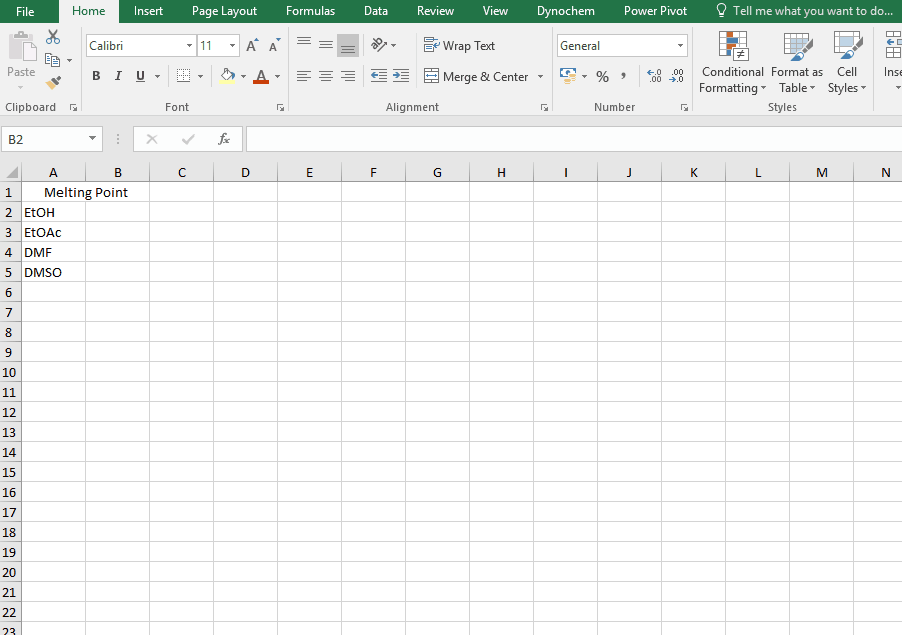List of Property codes

 Property code Property Property code Property Antoines Antoines FLVL Lower Flammability Limit % UNIFAC VLE Text showing UNIFAC VLE fragmentation FLTL Lower Flammability Limit Temperature UNIFAC LLE Text showing UNIFAC LLE fragmentation FLVU Upper Flammability Limit % UNIFAC Modified Text showing UNIFAC modified fragmentation FLTU Upper Flammability Limit Temperature CAS CAS Registry Number AIT Auto Ignition Temperature MW Molar mass HSUB Heat of Sublimation MI_MASS Monoisotopic mass ACEN Acentric Factor LINEAR_FORMULA Linear formula RG Radius of Gyration FORMATTED_LINEAR_FORMULA Formatted linear formula SOLP Solubility Parameter HILL_FORMULA Hill formula DM Diplole Moment FORMATTER_HILL_FORMULA Formatted Hill formula VDWV van der Waals Reduced Volume SMILES SMILES representation VDWA van der Waals Reduced Area InChI IUPAC international chemical identifier RI Refracrtive Index InChIKEY Hashed version of the full standard InChI PAR Parachor FAMILY Solvent family DC Dielectric constant RVALUE Relative Van der Waals volume, R Hilde Hildebrand solubility parameter QVALUE Relative Van der Waals surface area, Q HAND Hansen dispersion forces MP Melting Point at 1atm HANP Hansen polar forces NBP Normal Boiling Point HANH Hansen hydrogen bonds TPT Triple Point Temperature ICH_CLASS ICH Residual Solvent Class TPP Triple Point Pressure SOLVENT_TYPE Solvent Type including ACS Class TC Critical Temperature ACS_SAFETY ACS Greenness Safety PC Critical Pressure ACS_HEALTH ACS Greenness Health VC Critical Volume ACS_ENV_AIR ACS Greenness Env (Air) ZC Critical Compressibility Factor ACS_ENV_WATER ACS Greenness Env (Water) LVOL Liquid Molar Volume at 298.15 K ACS_ENV_WASTE ACS Greenness Env (Waste) HFOR Enthalpy of Formation for Ideal Gas GSK_CLASS GSK Guide 2009 Solvent Class GFOR Gibbs Energy of Formation for Ideal Gas GSK_FLAMM GSK Guide 2009 Flammability & Explosion ENT Absolute Entropy of Ideal Gas GSK_REACT GSK Guide 2009 Reactivity/Stability HSTD Standard State Enthalpy of Formation GSK_HEALTH GSK Guide 2009 Health GSTD Standard State Gibbs Energy of Formation GSK_WASTE GSK Guide 2009 Waste SSTD Standard State Absolute Entropy GSK_ENV_IMP GSK Guide 2009 Environmental impact HFUS Enthalpy of Fusion at Melting point GSK_LIFECYC GSK Guide 2009 Life Cycle Score HCOM Net Standard Enthalpy of Combustion GSK_LEGAL_FLAG GSK Guide 2009 Legislation Flag FP Flash Point GSK_EHS_FLAG GSK Guide 2009 EHS Red Flag

For further details about the ACS solvent selection guide, visit the following ACS link. The guide assigns a score from 1 to 10 for each solvent under the respective categories, with a score of 10 being of most concern and a score of 1 suggesting few issues.  For the ACS scores, 1=good and 10=bad.

For further details about the GSK solvent selection guide, visit the following link. Each solvent is scored from 1 (red) to 10 (green) to give a relative ranking for every solvent in the guide in each category, where the score is based on data or a physical observable property.  Supplementary information is available here. In the GSK scores, 1=bad and 10=good.

DC_GetTDepProperty function

The DC_GetTDepProperty allows you to search for temperature dependent properties for materials such as liquid density and liquid viscosity. In its simplest form it says:

• DC_GetTDepProperty("material name", "property code", temperature at which you want this property)

The resulting output for a DC_GetTDepProperty is the numerical value for that solvent property at that temperature.

Syntax

• DC_GetTDepProperty(“material”, “property code”, temperature

 Argument name Description Material (required) The material whose property you want Property (required) The material that you want Temperature (required) The temperature for the property

Example

Find the liquid density of EtOH at 25 C up to 50 C, in increments of 5 C.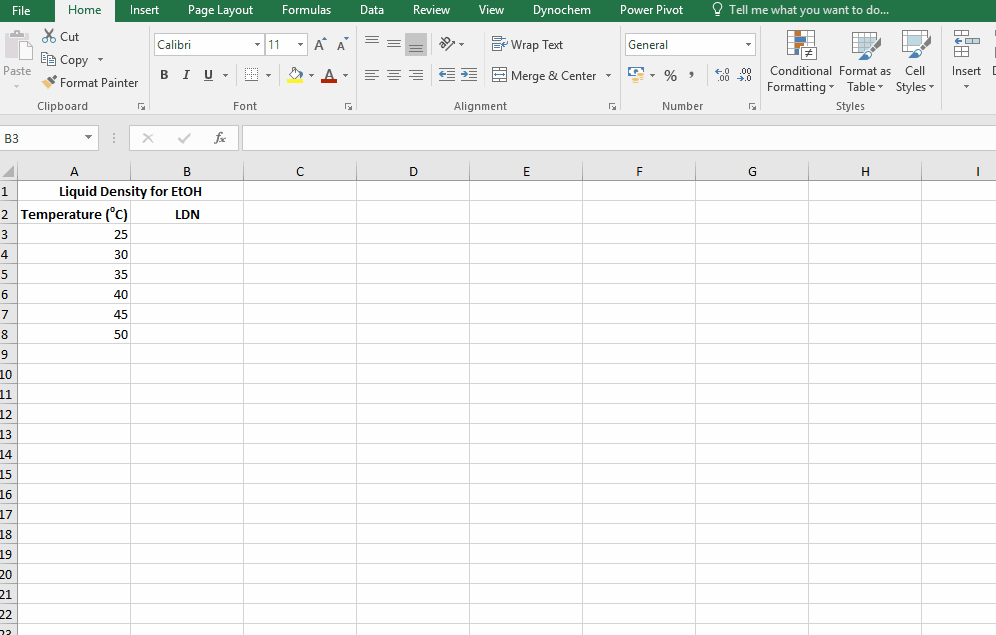List of Property codes

 Solvent Property Code Solvent Property Units LDN Liquid Density kg/m3 LCP Liquid Specific Heat Capacity kJ/kg.K LVS Liquid Viscosity mPa.s LTC Liquid Thermal Conductivity W/m.K LVP Vapor Pressure of the Liquid bar HVP Heat of Vaporization kJ/mol SDN Solid Density kg/m3 SCP Solid Specific Heat Capacity kJ/kg.K STC Solid Thermal Conductivity W/m.K VTC Vapor Thermal Conductivity W/m.K SVP Vapor Pressure of Solid bar ICP Ideal Gas Specific Heat Capacity kJ/kg.K SVR Second Virial Coefficient m3/kmol VVS Vapor Viscosity mPa.s SUT Surface Tension N/m

DC_MixtureBinary function

The DC_MixtureBinary function allows you to calculate temperature-dependent properties for binary solvent mixtures such as the liquid density or heat capacity.  Thermodynamic ideality is assumed when calculating these properties and components are assumed to be miscible.

Syntax

• DC_MixtureBinary("Property Code", "Temperature" , "Solvent1" , "wt% 1" , "Solvent2" , "wt% 2")

 Argument Description Property Code The property you want Temperature Real value. This is the temperature in degree Celsius Solvent 1 Solvent name (e.g. MeOH, EtOAc, Water) wt% 1 Real value. This is the weight % of the first solvent Solvent 2 Solvent name wt% 2 Real value. This is the weight % of the second solvent
DC_MixtureTernary function

The DC_MixtureTernary function allows you to search for temperature dependent properties for ternary solvent mixtures such as the liquid density or heat capacity.  Thermodynamic ideality is assumed when calculating these properties and components are assumed to be miscible.

Syntax

• DC_MixtureTernary("Property Code", "Temperature" , "Solvent1" , "wt% 1" , "Solvent2" , "wt% 2" , "Solvent3" , "wt% 3")

 Argument Description Property Code The property you want Temperature Real value. This is the temperature in degrees Celsius Solvent 1 Solvent name (e.g. MeOH, EtOAc, Water) wt% 1 Real value. This is the weight % of the first solvent Solvent 2 Solvent name wt% 2 Real value. This is the weight % of the second solvent Solvent 3 Solvent name wt% 3 Real value. This is the weight % of the third solvent

DC_ActivityBinary

The DC_ActivityBinary function calculates the activity coefficients for binary solvent mixtures.  Activity coefficients may be used to calculate behaviour in non-ideal systems.

Syntax

• DC_ActivityBinary("Method", "Temperature" , "Solvent1" , "Mole Fraction 1" , "Solvent2" , "Mole Fraction 2")

 Argument Description Method Method (NRTL, UNIQUAC, UNIFAC VLE, UNIFAC LLE, UNIFAC modified) Temperature Real value. This is the temperature in degree Celsuis Solvent 1 Solvent name (e.g. Meoh, EtOAc, Water) Mole Fraction 1 Real value. This is the mole fraction of the first solvent Solvent 2 Solvent name Mole Fraction 2 Real value. This is the mole fraction of the second solvent
DC_ActivityTernary

The DC_ActivityTernary function calculates the activity coeffiicent for ternary solvent mixtures.  Activity coefficients may be used to calculate behaviour in non-ideal systems.

Syntax

• DC_ActivityTernary("Method", "Temperature" , "Solvent1" , "Mole Fraction 1" , "Solvent2" , "Mole Fraction 2", "Solvent3" , "Mole Fraction 3")

 Argument Description Method Method (NRTL, UNIQUAC, UNIFAC VLE, UNIFAC LLE, UNIFAC modified) Temperature Real value. This is the temperature in degrees Celsius Solvent 1 Solvent name (e.g. MeOH, EtOAc, Water) Mole Fraction 1 Real value. This is the mole fraction of the first solvent Solvent 2 Solvent name (e.g. MeOH, EtOAc, Water) Mole Fraction 2 Real value. This is the mole fraction of the second solvent Solvent 3 Solvent name (e.g. MeOH, EtOAc, Water) Mole fraction 3 Real value. This is the mole fraction of the third solvent
DC_SourceBIPs

The DC_SourceBIPS function allows you to check the source of the binary interaction parameters (BIPs) used the the built-in Materials system when estimating activity coefficients.

Syntax

• DC_SourceBIPs("solvent one", "solvent two", "method")

 Argument name Description Solvent one (required) Name of the first solvent Solvent two (required) Name of the second solvent Method (required) NRTL/UNIQUAC

Outputs

• Regressed to data (DDB)
• Regressed to Mod UNIFAC
• Cannot find BIPs

Example:

To determine whether the following solvent pairs have BIPs in the database: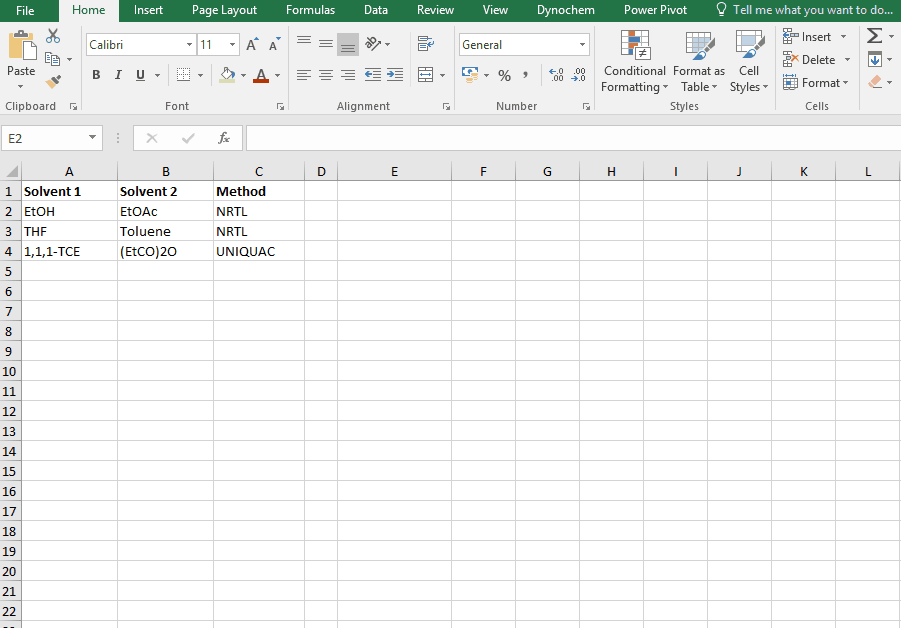DC_GetH2Henry

The DC_GetH2Henry allows you to search for the Henry constant for Hydrogen in (mol/m3)/(mol/m3).   The resulting output for a DC_GetH2Henry is the numerical value for that solvent property at that particular temperature.

The following references are used as sources to provide results from this function:

1 J. M. SHAW, A Correlation for Hydrogen Solubility in Alicyclic and Aromatic Solvents, THE CANADIAN JOURNAL OF CHEMICAL ENGINEERING. VOLUME 65. APRIL 1987.
2 "Thi-Kim-Hoang Trinh, Jean-Charles de Hemptinne, Rafael Lugo, Nicolas Ferrando and Jean-Philippe Passarello, Hydrogen Solubility in Hydrocarbon and Oxygenated Organic Compounds, J. Chem. Eng. Data 2016, 61, 19−34"
3 "The properties of gases & liquids” by Reid, Prausnitz and Poling (Fourth Edition, page332, section 8-11).
4 U. J. JÁUREGUI-HAZA, E. J. PARDILLO-FONTDEVILA, A. M. WILHELM and H. DELMAS, SOLUBILITY OF HYDROGEN AND CARBON MONOXIDE IN WATER AND SOME ORGANIC SOLVENTS, Latin American Applied Research 34:71-74 (2004).
5 Young, C. L. "Hydrogen and Deuterium" Solubliity Data Series Volume 5/6 Pergamon Press 1981

Syntax

• DC_GetH2Henry(“solvent”, temperature)

 Argument name Description Solvent (required) The solvent whose property you want Temperature (required) The temperature for the property

Example:

Find the Henry constant for Hydrohen in MeOH at 30 degrees.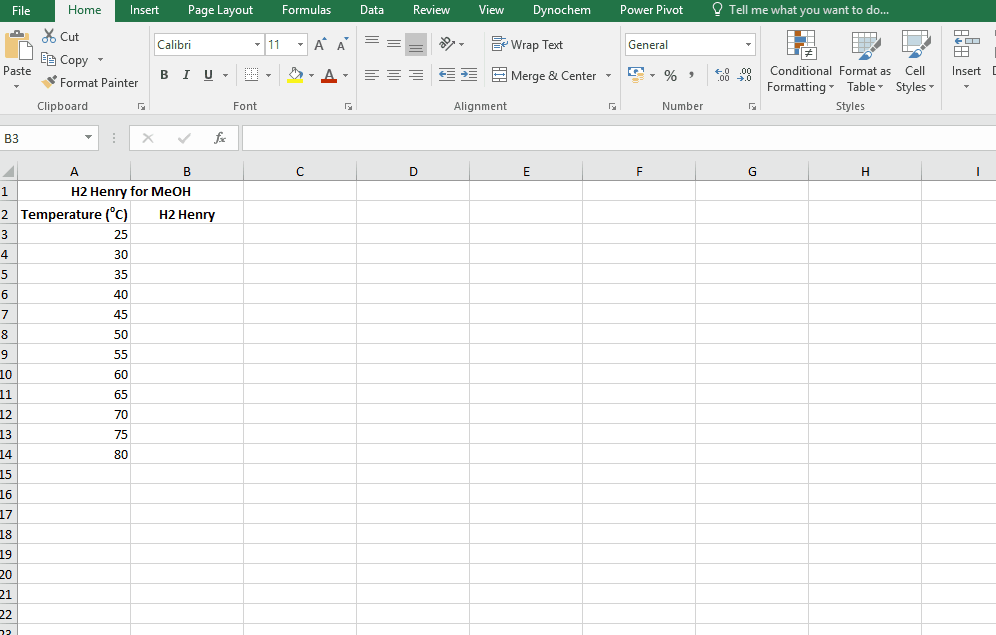DC_CalcMW

The DC_CalcMW allows you to calculate the molecular weight for a compound from a valid chemical formula.

Syntax

• DC_CalcMW("chemical formula")

Example:

Calculate the molar mass for the following compounds:

i. CH4

ii. Cl2C:

iii. Na[+]

iv. C2O4[2-]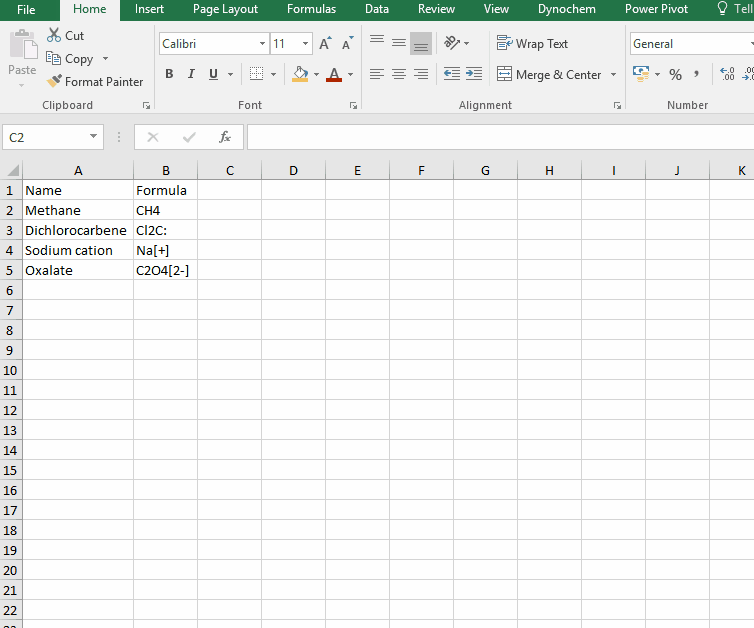DC_CalcMIMass

The DC_CalcMIMass allows you to calculate the monoisotopic mass for a compound from a valid chemical formula.

Syntax

• DC_CalcMIMass("chemical formula")

Example:

Calculate the monoisotopic mass for the following compounds:

i. CH4

ii. Cl2C:

iii. Na[+]

iv. C2O4[2-]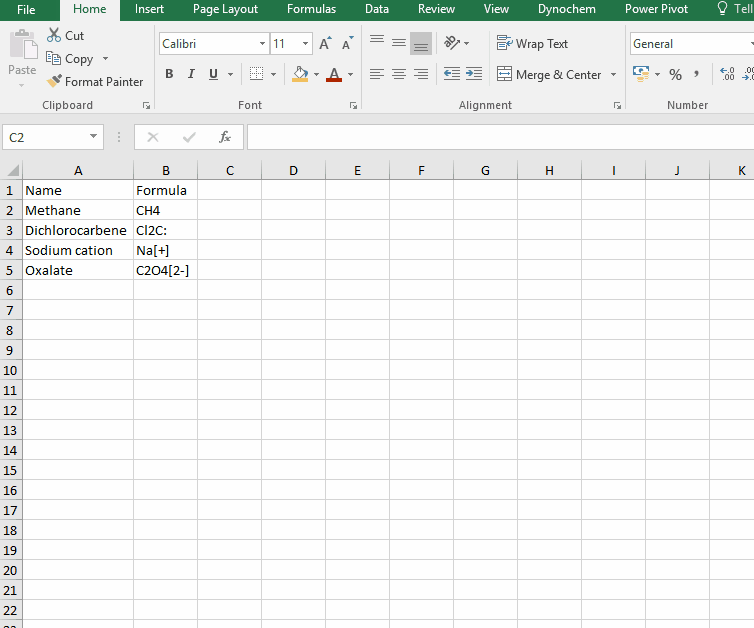DC_Format

The DC_Format function allows you to format the chemical formula where all the appropriate numbers are subscripted.

Syntax

• DC_Format(“chemical formula”)

For example:

The following examples show how various compounds can be formatted. For examples, if charged compounds are formatted, the charge is placed inside square bracket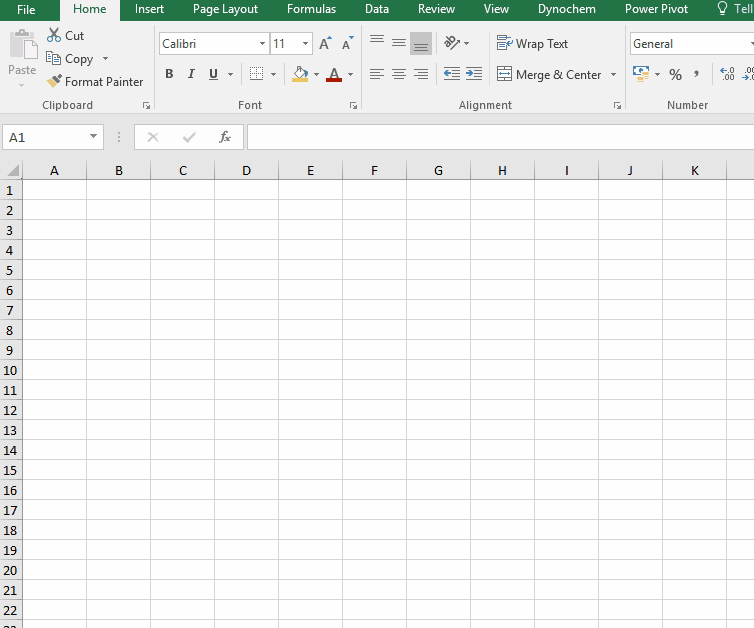DC_Visvalingam()

This function allows you to reduce your dataset using the Visvalingam-Whyatt algorithm.

Syntax

• DC_Visvalingam("Series", "Points per Series" , "Method")

 Argument Description Series Select a range of values to reduce Points per Series Enter a number. The data series will be reduced to this number of points. Method Options: Lead or Individual.  Lead will determine the retained points using a single series; Individual will choose retained points separately for each series.
DC_Regression()

Fits the parameters of a proposed model with predictors to response data using OLS or LASSO method

Syntax

• DC_Regression(Method, Factors, Responses, Features)

 Argument Description Method Options: OLS or LASSO. Alternatively you can type in "LASSO:MaxIt=1000; Nfold=4; Nlam=25" Factors These are the independent/explanatory variables Responses This is the response/dependent variable in your regression model Features This is a list containing the intercept coefficient, the predictors (x1, x2,...), transformations (e.g. log(x1), exp(x2)), polynomials (x2, x3), and/or interaction terms (x1.x2)

Output

 b0 This is the fitted intercept coefficient b1, b2,… These are the fitted slope coefficients RMSE Root mean square error. This is an estimate of the standard deviation of the error which is the average amount that the response deviates from the true regression line. R2 This is the coefficient of determination and is the proportion of variation explained by the model. Adjusted R2 The is the R2 value adjusted for the number of predictors in the model Model DoF This is model degrees of freedom Error DoF This is the error degrees of freedom F-statistic This is the value of the F-statistic. When this is larger than the critical F-value, the model is statistically significant Prob>F This is the p-value for the F-test. When this value is less than the chosen significance level, the model is statistically significant. SE This is the standard error for the slope and intercept coefficients t-statistic The test-statistic is used to determine whether the intercept or slope coefficients are statistically significant Prob>|t| param b0 This is the p-value. When this value is less than the significance level (α), then the parameters are statistically significant
DC_Prediction

The DC_Prediction function inputs explanatory values (your x values such as temperature, component amounts) into a regression model and returns the predicted values.

Syntax

• DC_Prediction(Factors, Model Definition, Features)

 Argument Description Factors This is a range that contains your explanatory/independent variables Model definition This is a range containing values of the intercept and slope coefficients Features This is a list containing the intercept coefficient and explanatory variables all separated by a semi-colon, e.g. y = b0; x1; x2
DC_ConvertUnit

The DC_ConvertUnit function allows you to convert values between different units, for example it can convert values in grams to pounds or centimeters to inches.

Syntax

• DC_ConvertUnit(number, "units in quotes", "units in quotes")

Examples:

Convert 30 cm into inches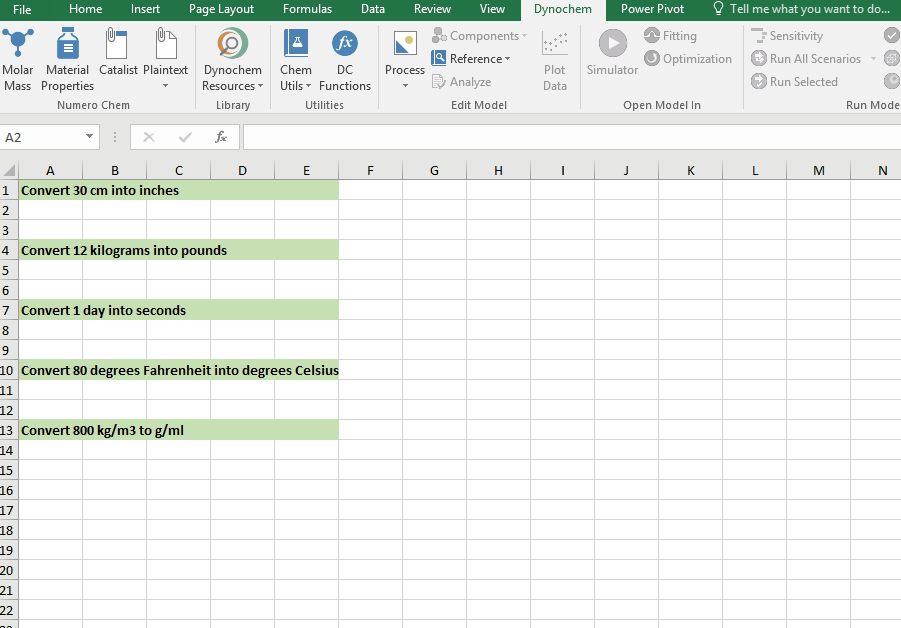Convert 12 kg into pounds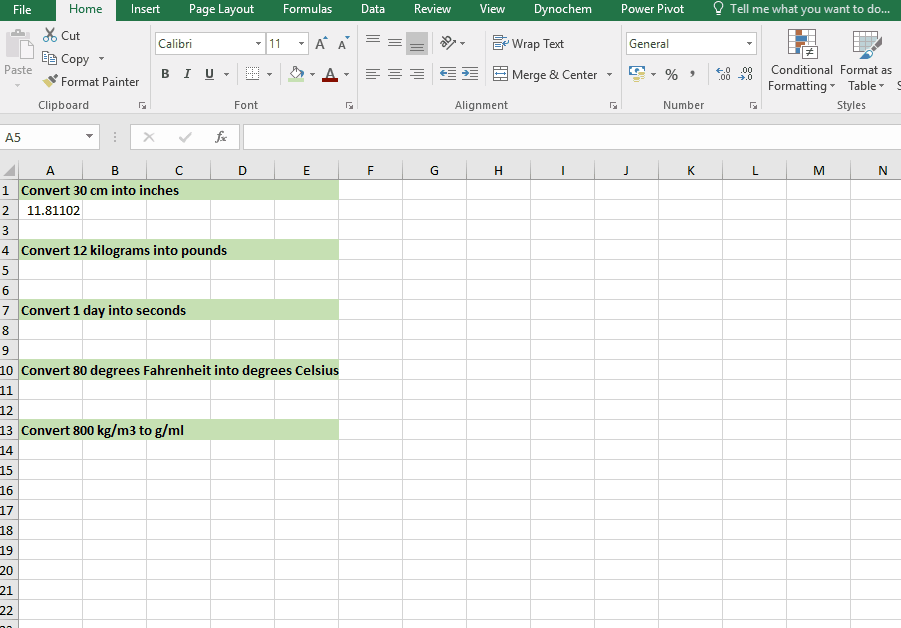Convert 1 day into seconds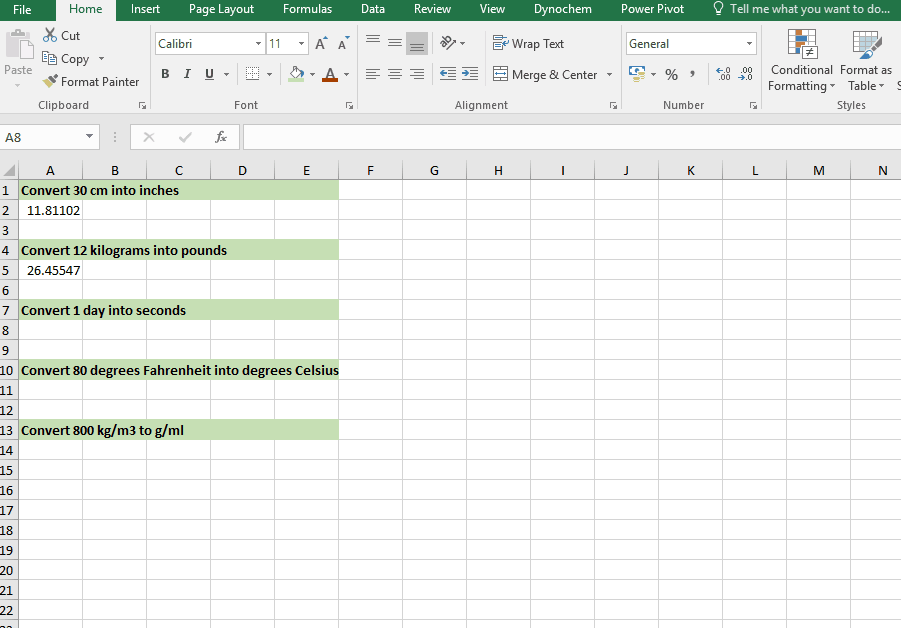Convert 80 degrees Fahrenheit into degrees Celsius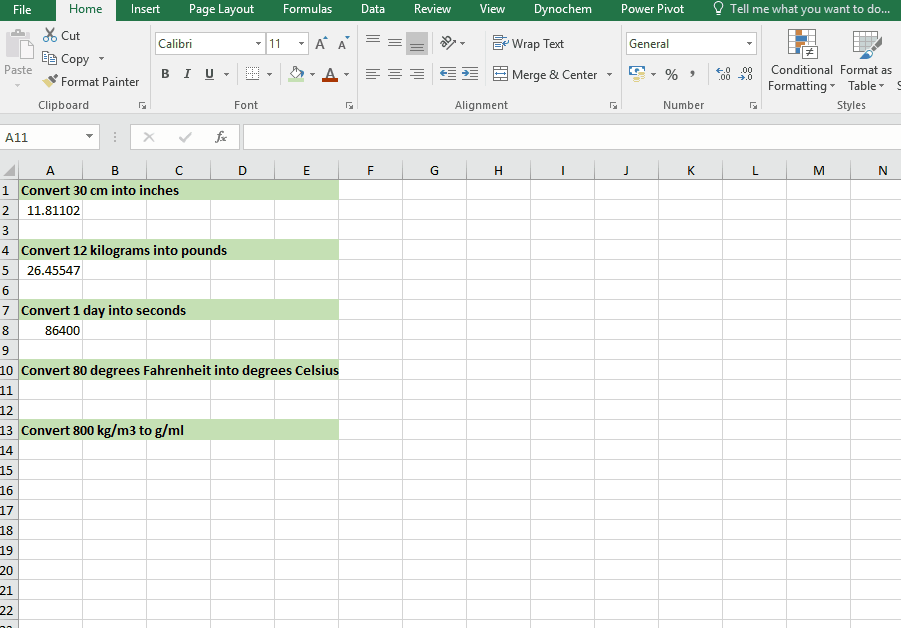Convert 998 kg/m3 into g/cm3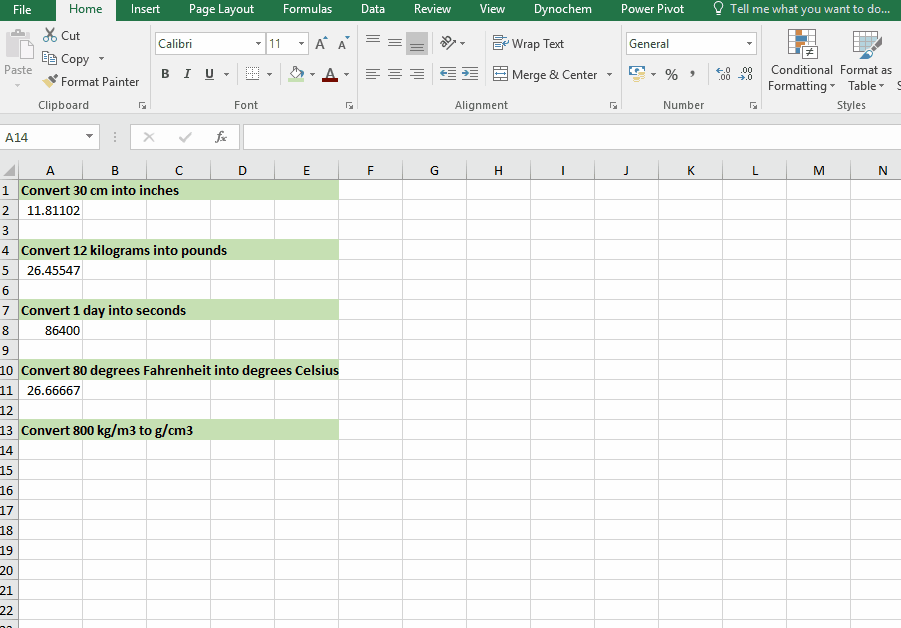DC_ConvertCurrency

This function returns the value for an amount converted from one currency to another, using real-time mid-market conversion rates.

Syntax

• DC_ConvertCurrency(Amount, From Currency, To Currency)

Convert 100 USD to EUR

1. First click on DC Functions in the Dynochem ribbon

2. Selct DC_Convert Currency from the list of DC functions available

3. Type in 100 in the Amount field, USD and then EUR as shown in the screenshot.

4. Click on cell A1, and press Insert.